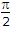# Electronics and Communication Engineering - Power Electronics

### Exercise :: Power Electronics - Section 3

6.

In a single phase dual converter, a1, and a2 are firing angles of the two converters. Then

 A. a1 - a2 =B. a1 + a2 = p C. a1 - a2 = p D. a1 + a2 =Explanation:

Since one converter is rectifying and the other is inverting

Vdc1 = -Vdc2

or a1 + a2 = p.

7.

The number of gates in an SCR is

 A. 1 B. 3 C. 4 D. 6

Explanation:

No answer description available for this question. Let us discuss.

8.

The waveshape of output voltage of half bridge inverter is

 A. sinusoidal B. square C. triangular D. either (a) or (b)

Explanation:

No answer description available for this question. Let us discuss.

9.

A 3 phase induction motor is controlled by voltage and frequency control such that the ratio V/f is constant. At 50 Hz, the speed is 1440 rpm. At 25 Hz, the speed is likely to be

 A. about 1350 rpm B. about 720 rpm C. about 360 rpm D. either (b) or (c)

Explanation:

In this case synchronous speed at 50 Hz is 1500 rpm. Therefore synchronous speed at 25 Hz is 750 rpm. Since slip is about 3 - 5%, speed is about 720 rpm.

10.

The gate current required to turn on an SCR is

 A. few amperes B. a few mA C. almost equal to anode current D. about 50% of anode current